##### Statistics: 1001 Practice Problems For Dummies (+ Free Online Practice)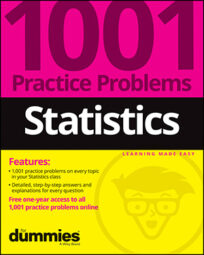Sample results vary — that's a major truth of statistics. You take a random sample of size 100, find the average, and repeat the process over and over with different samples of size 100. Those sample averages will differ, but the question is, by how much? And what affects the amount of difference?

Understanding this concept of variability between all possible samples helps determine how typical or atypical your particular result may be. Sampling distributions provide a fundamental piece to answer these problems.

Solve the following problems that introduce the basics of sampling distributions.

## Sample questions

1. Suppose that 10,000 students took the AP statistics exam this year. If you take every possible sample of 100 students who took the AP exam and find the average exam score for each sample and then put all those average scores together, what would it represent?

Answer: a sampling distribution of the sample means

A sampling distribution is a collection of all the means from all possible samples of the same size taken from a population. In this case, the population is the 10,000 test scores, each sample is 100 test scores, and each sample mean is the average of the 100 test scores.

2. A GPA is the grade point average of a single student. Suppose that you found the GPA for every student in a university and found that the mean of all those GPAs is 3.11. What statistical notation do you use to represent this value of 3.11?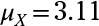Because you found the average GPA of every student in the university, you used a population value, which needs a Greek letter.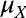refers to the mean of all individual values in the population.

3. If you roll two fair dice, look at the outcomes, and find the average value, you could get any number from 1 (where both your dice came up 1) to 6 (where both dice came up 6).

However, in the long run, if you took the average of all possible pairs of dice, you'd get 3.5 (because that's the average value of the numbers 1 through 6). How do you represent 3.5 in this situation using statistical notation?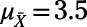Here, you take all possible samples (of the same size – in this case, of size 2), find all their possible means, and treat those as a population. Then, you find the mean of that entire population of sample means. The notation for this is4. Suppose that you roll several ordinary six-sided dice, choose two of those dice at random, and average the two numbers. Suppose the average of these two dice is 3.5.

How would you express the 3.5 in statistical notation?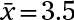Because the value is the result of only a sample of dice rolls, and not the full population of all possible rolls, you must use the sample mean notation.

5. Which of the following would not ordinarily be considered a sampling distribution?

(A) a distribution showing the average weight per person in several hundred groups of three people picked at random at a state fair

(B) a distribution showing the average proportion of heads coming up in several thousand experiments in which ten coins were flipped each time

(C) a distribution showing the average percentage daily price change in Dow Jones Industrial Stocks for several hundred days chosen at random from the past 20 years

(D) a distribution showing the proportion of parts found to be deficient in each of several hundred shipments of parts, each of which has the same number of parts in it

(E) a distribution showing the weight of each individual football fan entering a stadium on game day

Answer: E. a distribution showing the weight of each individual football fan entering a stadium on game day

A sampling distribution is a population of data points where each data point represents a summary statistic from one sample of individuals. A population distribution is a population of data points where each data point represents an individual.

If you need more practice on this and other topics from your statistics course, visit 1,001 Statistics Practice Problems For Dummies to purchase online access to 1,001 statistics practice problems! We can help you track your performance, see where you need to study, and create customized problem sets to master your stats skills.